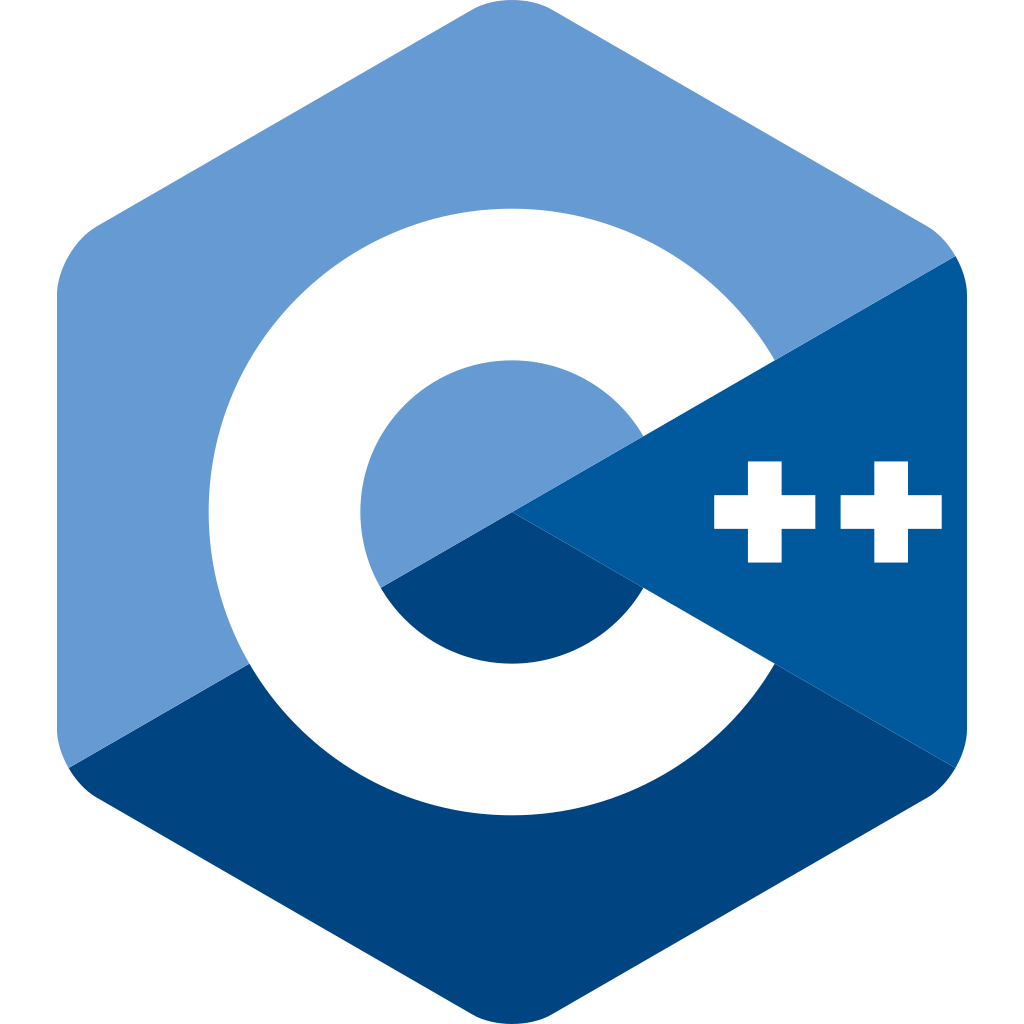blog

# C++: Disable implicit conversion in specific contexts only

#### You get one implicit conversion, so burn it with a wrapper

Lawrence Murray on 14 November 2023

Consider the following class template:

``````template<class T>
struct A {
template<class U>
A(const A<U>& other);
};
``````

This enables conversion between objects of type `A<T>` and `A<U>` when `T` and `U` are different. Perhaps `A` is a container class, for example, and we wish to enable conversion between `A<int>` and `A<float>` among other things.

The following will compile:

``````A<float> f();
A<int> i();
A<float> g() {
return i();
}
``````

While `i()` returns an `A<int>`, it can be implicitly converted to an `A<float>`, and so this is allowed.

But perhaps conversion between these types is expensive, and we want to ensure that the programmer really wants to do so before it is triggered. We might disallow implicit conversion by declaring the `A()` constructor to be `explicit`:

``````template<class T>
struct A {
template<class U>
explicit A(const A<U>& other);
};
``````

The above code will now fail to compile with a message such as the following:

``````> g++ -c test.cpp
test.cpp: In function ‘A<float> g()’:
test.cpp: error: could not convert ‘i()’ from ‘A<int>’ to ‘A<float>’
11 |   return i();
|          ~^~
|           |
|           A<int>
``````

We could make this work again by using explicit conversion, i.e. replacing `return i()` with `return A<float>(i())`. The explicit constructor permits such explicit conversion, but forbids the previous implicit conversion.

But using an explicit constructor affects all usage of `A`. Perhaps we wish the implicit conversion to be allowed in general, but not in some contexts, or perhaps we want to ensure that some particular code that we have written is not doing a copy when it was not intended. For this, we will need to remove the `explicit` again, but can create a sort of wrapper type utility:

``````template<class T>
struct nocopy {
const T& ref;
nocopy(const T& ref) : ref(ref) {
//
}
operator const T&() const {
return ref;
}
};
``````

This takes (and stores) a reference to an object of some other type. It also has a user-defined conversion function (also known as a cast operator) that returns the original reference it is given.

Now we see the following:

``````A<float> f();
A<int> i();
A<float> g() {
return nocopy(f());  // works
}
A<float> h() {
return nocopy(i());  // does not work!
}
A<float> j() {
return f();  // works!
}
A<float> k() {
return i();  // works!
}
``````
``````> g++ -c test.cpp
test.cpp: In function ‘A<float> h()’:
test.cpp: error: could not convert ‘nocopy<A<int> >(i())’ from ‘nocopy<A<int> >’ to ‘A<float>’
25 |   return nocopy(i());  // does not work!
|          ^~~~~~~~~~~
|          |
|          nocopy<A<int> >
``````

That is, whenever `nocopy` is used we effectively disable implicit conversion; this is why `h()` fails to compile but all the other functions succeed. We might use `nocopy` as a sort of static assertion to ensure correctness whenever we have implemented a function that should not result in a copy on return.

This approach works by relying on the rules for implicit conversion (quoting, emphasis mine):

Implicit conversion sequence consists of the following, in this order:

1. zero or one standard conversion sequence;
2. zero or one user-defined conversion;
3. zero or one standard conversion sequence (only if a user-defined conversion is used).

The one user-defined conversion allowed is burned by the `nocopy` wrapper, so that the user-defined conversion operator in `A` cannot be used.

Complete code for further play:

``````template<class T>
struct A {
template<class U>
A(const A<U>& other);
};

template<class T>
struct nocopy {
const T& ref;
nocopy(const T& ref) : ref(ref) {
//
}
operator const T&() const {
return ref;
}
};

A<float> f();
A<int> i();

A<float> g() {
return nocopy(f());  // works
}
A<float> h() {
return nocopy(i());  // does not work!
}
A<float> j() {
return f();  // works!
}
A<float> k() {
return i();  // works!
}
``````
blog Related?
##### GPU Programming in the Cloud

A how-to and round-up of cloud service providers.

Lawrence Murray

22 Nov 22

blog Related?
##### Combinatorial instantiation of C++ templates with std::variant

An alternative to explicit instantiations and macros.

Lawrence Murray

11 Jun 23

blog Related?
##### Sums of Discrete Random Variables as Banded Matrix Products

Zero-stride catch and a custom CUDA kernel.

Lawrence Murray

16 Mar 23

photography Previous
1

3 Sep 23